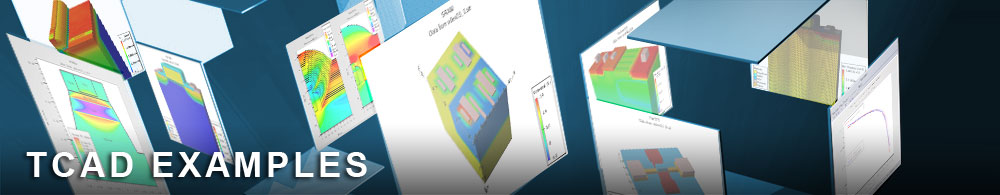Mode Space Basis Generation

## vaex02.in : Mode Space Basis Generation

Requires: Victory Atomistic
Minimum Versions: Victory Atomistic 1.0.1.R

This example demonstrates the generation of a mode space basis based on G. Milnikov, N. Mori, and Y. Kamakura, "Equivalent transport models in atomistic quantum wires," Phys. Rev. B, vol. 85, no. 3, p. 035317, Jan. 2012. It shows:

1. Solution of band initial band structure. 2. Solution of reduced band structure through various iterations of Milnikov method.

The basis is constructed for a silicon nanowire with the dimensions of 4-by-4 unit cells (2.172 nm by 2.172 nm), and the initial band structure calculations are solved on a Hamiltonian in the sp3d5s* tight-binding basis. The basis can be used for any nanowire with this cross-sectional area and any length.

The device contains three domains, SuperCell, SuperCell2 and SuperCell3, of which SuperCell is the primary domain for which the basis is solved and the other two are used for obtaining coupling Hamiltonians. The first actions performed by this input deck are the output of these structures to VTK files for visualization.

The band structure is calculated in the 10-band sp3d5s* basis using information from the Schroedinger solver {Bold} Cbands. Note that the {Bold} Cbands2 Schroedinger solver is needed for solving the coupling in the bandstructure calculation.

The {Bold} Test_MS solver (solver type ModeSpace) is the primary solver in which most mode-space-related parameters can be tweaked. The following are options of highest interest:

{Bold} N_try_eleminate determines the maximum number of iterations for obtaining a minimum basis. For each iteration, the screen output will show "[ModeSpace]: number of new ka points for optimization = <n>" where <n> is the k-points that were added during the iteration by adding an artificial dimension to the reduced Hamiltonian as in Milnikov 2012. Keep in mind that this number does not have to reach 0 for the basis to be usable. It is recommended that one compare the reduced bandstructure (using outputs K_Reduced_BS.dat and E_Reduced_BS.dat) to the full bandstructure (outputs K_Init_BS.dat and E_Init_BS.dat) to see if it is viable for electron transport.

{Bold} auto_energy_window, when set to true, activates the options delta_Ec, delta_Ev and delta_Eg. These options set the desired energy window into the conduction band, valence band, and bandgap respectively. This desired energy window is the energy range which the mode space algorithm will attempt to match to the original basis band structure. Alternatively, when auto_energy_window is set to false, the options EL and EH can be set, as the bottom and top limits respectively, of the desired energy range. It is important to consider that solving a larger energy window will result in physical results for that entire energy, but will require more time to compute and may result in a less significant basis reduction.

The output for this example includes vtk structure output, initial band structure data (E_Init_BS.dat and K_Init_BS.dat), reduced band structure data (E_Reduced_BS.dat and K_Reduced_BS.dat), and the resulting basis which can be used for basis reduction using the LRAModule solver (Unitary_matrix.dat). The example generates the values of Unitary_matrix.dat.

Visualization: 1. vtk structure output consists of the device structure. {Bold} Use Paraview to visualize these structure files (.vtk extension).

2. band structure data files : Files required to generate E-K plots. {Bold} Use a graph plotter such as Matlab, Python plotter, or Origin to plot the E-k data files.

To load and run this example, select the Load button in DeckBuild > Examples. This will copy the input file and any support files to your current working directory. Select the Run button in DeckBuild to execute the example.